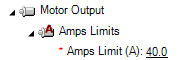Motor current

The controller is constantly monitoring and reporting the motor's current, by using its integrated current sensors. This current can be viewed through the Motor amps parameter, that reports the RMS current of the motor, calculated every millisecond.The current of the motor depends on the applied PWM voltage, the motor specs and the motor speed and can be described for each phase with the following equation:

V = R*i + L*(di/dt) + e,

where V is the applied voltage, R and L are the phase resistance and inductance and e is the motor back emf at a given speed. Different motors will consume different current and go at different speeds by applying the same PWM voltage to them.

In the basic operating modes of the controller, where the Torque Loop is disabled, the motor current will be directly linked to the speed demand. In such scenarions, the motor current can easily reach the maximum current that the motor can pull, and potentially cause damage to the controller.

Amp limiting function

The Roboteq controller is equipped with a safety feature that prevents the motor current from exceeding dangerous levels. This function is activated by configuring the "amp limit" parameter, which sets the maximum allowable value for the motor current. In cases where the motor attempts to draw more current than the limit, the controller will reduce its PWM voltage to prevent it from exceeding the threshold. However, this reduction in PWM voltage will have an impact on the motor's performance.The "amp limit" function can be employed to safeguard either the controller or the motor. If the motor has the capability to draw more current than the maximum current that the controller can deliver, then the "amp limit" parameter should be set to equal the maximum current capacity of the controller. Conversely, if the controller can deliver more current than the maximum capacity of the motor, then the "amp limit" parameter should be set to equal the maximum current that the motor can handle.

For example, suppose a controller has a maximum current capacity of 60 amps, but the motor's peak current is only 40 amps. In this case, the "amp limit" parameter should be set to 40 amps to prevent the motor from being damaged by excessive current.On the other hand, if the motor has a maximum current capacity of 75 amps, but the controller can deliver up to 60 amps, then the "amp limit" parameter should be set to 60 amps to protect the motor from overload. To ensure optimal protection, it is recommended to set the "amp limit" to a value lower than the maximum capacity of the controller, as the amp limiting function may not respond immediately due to its time response.Amps trigger function

The peak current of the motor or the controller is not intended to be consumed continuously and is typically utilized during the motor's acceleration and deceleration phases. The datasheet of the controller or motor specifies the maximum duration during which peak current can be utilized. Consuming peak current beyond the allowed time can result in permanent damage to the motor or controller.

Roboteq controller has a feature that can trigger an action if the motor current exceeds a threshold for longer than a defined period of time. This functionality can help in identifying erroneous conditions in which the motor malfunctions and consumes a higher current than usual for an extended period.The duration for which the action is triggered should be less than the maximum permissible time for the peak current. This may not be very straightforward at first, but consider the following scenario: suppose the "amps trigger delay" is set to 3 seconds. If the motor runs for 2.5 seconds and then stops, and then after one second the process is repeated, this can eventually lead to motor or controller overheating without the "amps trigger" function protecting it.

In the below example we have configured the amps limit to 55 A, alhtough peak current of the controller is 60 A, to provide headroom to the amp limit function to act. The amps trigger delay has been set to 3 sec, to allow this current during motor transnients (acceleration/deceleration) which typically will take less than that. Although the controller can withstand this peak current for up to 10 sec, the amps trigger function is set to a lower time due to the possibility of accumulated heat